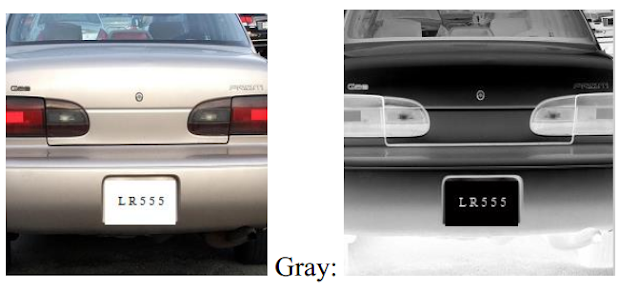# Verilog License Plate Recognition on FPGA

## A plate license recognition system is implemented in Matlab and then it is implemented on FPGA Xilinx Spartan-6 using Verilog. Below is the test environment for the system on FPGA.Image memory: 10 images and convert it into .dat format ( gray data). We use \$readmemh(synthesizable) Verilog command to initialize the memory by reading gray data from .dat file. 10 images are converted into Gray format and write to the .dat file by using Matlab.

#### Plate License Recognition core: Use a switch on FPGA as the “start” signal to trigger this core working and output the plate license numbers.

Monitor on LEDs: When the “done” signal is rising-edge, this block periodically displays plate license numbers.### Top level Verilog code for testing the license plate recognition system:

```// fpga4student.com FPGA projects, Verilog projects, VHDL projects
// Verilog project: License Plate Recognition in Verilog and Matlab
// Top level module for testing the license plate recognition system
module Test_top(input clk // 33MHz
,rst, start,
output reg[5:0] led
);
reg [7:0] image_pixel_val;
// Outputs
wire done;  // fpga4student.com FPGA projects, Verilog projects, VHDL projects
reg[5:0] num1,num2,num3,num4,num5,num6,num7;
reg [2:0] count,count_4s;
wire CharCheck;
reg clk_4s,display;
integer counter;
reg [7:0] image_inv [0:65535];
// Instanitate the license plate recognition system
LPChRec uut (
.clk(clk),
.rst(rst),
.start(start),
.image_pixel_val(image_pixel_val),
.CharCheck(CharCheck),
.done(done)
);
initial begin
end
always @(posedge clk or posedge rst) // clock 4s
begin
if(rst) begin
clk_4s <= 1'b0;
counter <= 0;
end
else
begin
counter <= counter + 1;
if(counter <= 66000000) clk_4s <= 1'b0;
else if(counter > 132000000)
begin
counter <=0;
end
else
clk_4s <= 1'b1;
end
end
always @(posedge clk)
begin
end
// fpga4student.com FPGA projects, Verilog projects, VHDL projects
always @(posedge clk or posedge rst)
begin
if(rst) begin
count = 3'd0;
num1 <= 40;
num2 <=0;
num3 <= 0;
num4 <= 0;
num5 <= 0;
num6 <= 0;
display <= 0;
end
else
begin
if(CharCheck) begin
count = count + 1'd1;
if(count==3'd1)
else if(count==3'd2)
else if(count==3'd3)
else if(count==3'd4)
else if(count==3'd5)
else if(count==3'd6)
else if(count==3'd7)
else begin
num1 <= 0;
num2 <=0;
num3 <= 0;
num4 <= 0;
num5 <= 0;
num6 <= 0;
end
end
if(done) display <= 1;
end
end
// fpga4student.com FPGA projects, Verilog projects, VHDL projects
always @(posedge clk_4s or posedge rst) begin
if(rst) begin count_4s <= 0; led <=0; end
else begin
if(display) begin
count_4s <= count_4s + 1;
if(count_4s==0) led <= num1;
else if(count_4s==1) led <=num2;
else if(count_4s==2) led <=num3;
else if(count_4s==3) led <=num4;
else if(count_4s==4) led <=num5;
else if(count_4s==5) led <=num6;
else if(count_4s==6) led <=num7;
else count_4s <= 0;
end
end
end
endmodule
```

### Verilog code for License Plate Recognition System:

```// fpga4student.com FPGA projects, Verilog projects, VHDL projects
// Verilog project: License Plate Recognition in Verilog
// Top level Verilog code for License Plate Recognition
module LPChRec(
input clk,
input rst,
input start,
input [7:0] image_pixel_val,
output CharCheck,
output done
);
wire [7:0] ccl_th_low;
wire [7:0] ccl_th_high;
wire [7:0] image_pixel_val1;
wire [7:0] ImgVal;
wire [55:0] ObjInfo;
wire                ccl_done,active;
assign ccl_th_low = 8'd40;
assign ccl_th_high = 8'd255;
assign image_pixel_val1 = image_pixel_val;
assign ImgVal                    = image_pixel_val;
// fpga4student.com FPGA projects, Verilog projects, VHDL projects
// Image processor unit
image_processor image_processor_inst (
.image_pixel_val(image_pixel_val1),
.clk(clk),
.rst(rst),
.ccl_start(start),
.ccl_th_low(ccl_th_low),
.ccl_th_high(ccl_th_high),
.ccl_done(ccl_done),
.ccl_mem_result_data(ObjInfo)
);
// fpga4student.com FPGA projects, Verilog projects, VHDL projects
// Create object module
CreateObj CreateObjInst (
.clk(clk),
.rst(rst),
.start(ccl_done),
.thresh(ccl_th_low),
.ObjInfo(ObjInfo),
.ImgVal(ImgVal),
.CharCheck(CharCheck),
.active(active),
.done(done)
);
endmodule
```

### Matlab Recognition Results:#### Video Demo for the License Plate Recognition System on FPGA:

1.Hello,
I am interested in replicating this FPGA project and was wondering if you can provide some code and documentation that I can use. Thank you for your time it is very much appreciated.

thanks,

Roger C.

1.2.I am doing this same project for final year.Can u plz provide me the code and steps for implementing it.

3.2.Do we need any separate program for character recognition and Detection?

3.We need many more others code for recognition. Not only the code above.

4.Do you have all the codes to implement this on fpga?If yes,can you tell me how to get it?

5.does anyone have the complete code for this project? will the above code work for the number plate recognition project?

6.Please send me that code, this code is not complete

1.Hi, this is just an example code for reference. Full code for this project is not provided.

2.I request to all members please provide a full code of this project and tell where I get this full code because I need this code for my final project

3.This project is complicated and it takes time to do. Try to spend some time on it to learn even more.